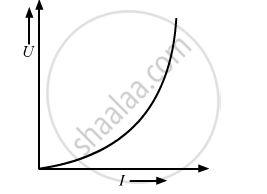# Plot a Graph Showing the Variation Of Magnetic Potential Energy Stored Versus the Current. - Physics

The current flowing through an inductor of self inductance L is continuously increasing. Plot a graph showing the variation of Magnetic potential energy stored versus the current.

#### Solution

Since magnetic potential energy is given by U =1/2LI^2Concept: Inductance - Mutual Inductance
Is there an error in this question or solution?